# Hardest colleges to get into in Wisconsin

Top 10 colleges in Wisconsin with the lowest acceptance rates
What are the hardest colleges to get into in Wisconsin? We've got you covered. We've compiled a national college database and have created a list of the hardest universities to get into in Wisconsin below. These are the hardest 4 year colleges to get into in Wisconsin, and sometimes some of the hardest colleges to get into in the US. You could even say these are the best colleges in Wisconsin. We also include each college's average SAT and ACT scores so that you can see where you're most competitive. Read on to find out more.

## Silver Lake College of the Holy Family acceptance rate

Silver Lake College of the Holy Family acceptance rate is 50%.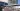The average SAT score for Silver Lake College of the Holy Family is 1010.

• SAT composite: 1010
• SAT math: 500

The average ACT score for Silver Lake College of the Holy Family is 17.

## University of Wisconsin Madison acceptance rate

University of Wisconsin Madison acceptance rate is 51.7%.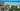The average SAT score for University of Wisconsin Madison is 1390.

• SAT composite: 1390
• SAT math: 725

The average ACT score for University of Wisconsin Madison is 30.

## Northland College acceptance rate

Northland College acceptance rate is 61.2%.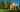The average SAT score for Northland College is 1170.

• SAT composite: 1170
• SAT math: 570

The average ACT score for Northland College is 23.

## Lawrence University acceptance rate

Lawrence University acceptance rate is 62.5%.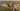The average SAT score for Lawrence University is 1340.

• SAT composite: 1340
• SAT math: 670

The average ACT score for Lawrence University is 29.

## Viterbo University acceptance rate

Viterbo University acceptance rate is 62.5%.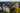The average SAT score for Viterbo University is 1140.

• SAT composite: 1140
• SAT math: 570

The average ACT score for Viterbo University is 23.

## Milwaukee School of Engineering acceptance rate

Milwaukee School of Engineering acceptance rate is 62.8%.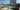The average SAT score for Milwaukee School of Engineering is 1260.

• SAT composite: 1260
• SAT math: 660

The average ACT score for Milwaukee School of Engineering is 27.

## Alverno College acceptance rate

Alverno College acceptance rate is 67.1%.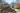The average SAT score for Alverno College is 1040.

• SAT composite: 1040
• SAT math: 520

The average ACT score for Alverno College is 19.

## Carthage College acceptance rate

Carthage College acceptance rate is 67.8%.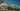The average SAT score for Carthage College is 1140.

• SAT composite: 1140
• SAT math: 580

The average ACT score for Carthage College is 24.

## University of Wisconsin Oshkosh acceptance rate

University of Wisconsin Oshkosh acceptance rate is 67.8%.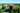The average SAT score for University of Wisconsin Oshkosh is 1110.

• SAT composite: 1110
• SAT math: 555

The average ACT score for University of Wisconsin Oshkosh is 22.

## Carroll University acceptance rate

Carroll University acceptance rate is 70.8%.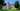The average SAT score for Carroll University is 1180.

• SAT composite: 1180
• SAT math: 590